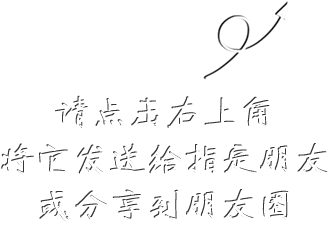12# 耳鼻咽喉医生临床随笔

## ¥ 46.4058.00现货已售出87件

1.换一个角度看医学，借助"随笔"这样一种雅俗共赏的形式，把医学的深奥道理，医者的点滴体会，以及与医学密切相关的其它事项（如科研与学术，医生与患者等），以一种轻松自如的口吻呈现给读者，唤起读者的共鸣，为推动医学进步而努力。
2.您支付成功后，我们在3个工作日内尽快为您发货。默认寄送方式为快递。
3.请务必核实您的姓名，电话，地址等信息，地址填写请具体到门牌号或者医院科室。
4.如需发票，请填写并提交信息。发票项目：杂志费。当月订单当月开发票，过时不补。请知悉。
5.投诉客服：010-51322386/2387/2388

• 删除
• 删除
• 删除
• 删除
• 删除
• 删除
• 删除
• 删除
• 删除
• 删除
• 删除
• 删除
• 删除
• 删除
• 删除
• 删除
• 删除
• 删除
• 删除
• 删除
• 删除
• 删除
• 删除
• 删除
• 删除
• 删除
• 删除
• 删除
• 删除
• 删除
• 删除
• 删除
• 删除
• 删除
• 删除
• 删除
• 删除
• 删除
• 删除
• 删除
• 删除
• 删除
• 删除
• 删除
• 删除
• 删除
• 删除
• 删除
• 删除
• 删除
• 删除
• 删除
• 删除
• 删除
• 删除
• 删除
• 删除
• 删除
• 删除
• 删除
• 删除
• 删除
• 删除
• 删除
• 删除
• 删除
• 删除
• 删除
• 删除
• 删除
• 删除
• 删除
• 删除
• 删除
• 删除
• 删除
• 删除
• 删除
• 删除
• 删除
• 删除
• 删除
• 删除
• 删除
• 删除
• 删除
• 删除
• 删除
• 删除
• 删除
• 删除
• 删除
• 删除
• 删除
• 删除
• 删除
• 删除
• 删除
• 删除
• 删除
• 删除
• 删除
• 删除
• 删除
• 删除
• 删除
• 删除
• 删除
• 删除
• 删除
• 删除
• 删除
• 删除
• 删除
• 删除
• 删除
• 删除
• 删除
• 删除
• 删除
• 删除
• 删除
• 删除
• 删除
• 删除
• 删除
• 删除
• 删除
• 删除
• 删除
• 删除
• 删除
• 删除
• 删除
• 删除
• 删除
• 删除
• 删除
• 删除
• 删除
• 删除
• 删除
• 删除
• 删除
• 删除
• 删除
• 删除
• 删除
• 删除
• 删除
• 删除
• 删除
• 删除
• 删除
• 删除
• 删除
• 删除
• 删除
• 删除
• 删除
• 删除
• 删除
• 删除
• 删除
• 删除
• 删除
• 删除
• 删除
• 删除
• 删除
• 删除
• 删除
• 删除
• 删除
• 删除
• 删除
• 删除
• 删除
• 删除
• 删除
• 删除
• 删除
• 删除
• 删除
• 删除
• 删除
• 删除
• 删除